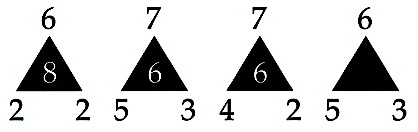### #20. Pattern Recognition Brain Teaser — The Empty Triangle

Please enjoy this brain teas­er com­pli­ments of puz­zle mas­ter Wes Car­roll.

The Emp­ty Tri­an­gleQues­tion:
Which fig­ure should be placed in the emp­ty tri­an­gle?

This puz­zle works your exec­u­tive func­tions in your frontal lobes by using your pat­tern recog­ni­tion, hypoth­e­sis test­ing, and log­ic. Let us know how you do!

3

SOLUTION:

The top num­ber minus the bot­tom left-hand num­ber is mul­ti­plied by the bot­tom right-hand num­ber to give the num­ber inside the tri­an­gle.

Next brain teas­er in Sharp­Brains’ top 25 series:

### 54 Responses

1.rob says:

i came up with the same answer as kevin the sums added across all equal 26 if 6 is placed in the tri­an­gle.

2.Toffee says:

Anoth­er option if you look at it as a set…
(6–2)+ (6–2) = 8
(7–5) +(7–3) = 6

For the third one and fourth as a set fol­low the same rule

(7+4)- (7–2)= 6
There­fore
(6+5) — (6–3) = 8

3.Dan Bowles says:

The key to the prob­lem is that it is pat­tern recog­ni­tion — that is that each tri­an­gle is a dis­tinct event.

4.david says:

#1 (6–2)*2=8
#2 (7–5)*3=6
#3 (7–4)*2=6
#4 (6–5)*3=

5.NightLord says:

I got 8.
First tri­an­gle total is 18.
Sec­ond tri­an­gle is 21.
Third tri­an­gle is 19.
This, with a skip­ping pat­tern the fourth tri­an­gle should jump to 22.
Thus, 8 in the last tri­an­gle.

6.Yomanda says:

3. fo sho.

7.zeda says:

(6*2)-(2*2)= 8
(7*3)-(5*3)= 6
(7*2)-(4*2)= 6
(6*3)-(5*3)= 3

I muli­ti­plied the top num­ber with the bot­tom right numbe. Then mul­ti­plied the bot­tom two num­bers and sub­tract­ed the two totals. Guess i took the longer route!

8.yahobahne says:

i say 7 is the miss­ing num­ber
pat­tern: 18,21,19,21…
21 is the con­stant num­ber while the oth­ers decrease by one

9.siobhan says:

6–2=4*2=8
7–5=2*3=6
7–4=3*2=6
6–5=1*3=3

10.Aaron says:

I got 8, just because it goes “6, 7, 7, 6” on top, then “8, 6, 6, X” in the tri­an­gle, so the pat­tern would make sense if it were 8.

11.sudhasini says:

IN first train­gle 8/2=4
IN sec­ond one
6/3=2+5=7
IN 3rd tri­an­gle
6/2=3+4=7
IN 4th tri­an­gle
3/3=1+5=6

12.Richard says:

1 3 5 7 9 R
whelmint

13.Lmnop says:

I haven’t checked the answer yet, but I’m going to go ahead and say that it’s 3.

14.Lmnop says:

After check­ing the way you were “sup­posed” to get to the answer, I found out I did it the “wrong” way. I did it the same way as zeda. I sup­pose the oth­er way would have been quick­er; oh well.

15.Zobi says:

I get 1

16.Truck says:

I get 2 as (7–5)*3 — 4 = 2

17.jumbo says:

In 1st tri­an­gle :6*2–2*2 =inside num­ber

In 2nd tri­an­gle :7*3–3*5 =inside num­ber
same for 3rd tri­an­gle
hence in last tri­an­gle num­ber is 3

18.Theresa says:

Wow, this works at least 3 oth­er ways. Oth­er peo­ple men­tioned two already but i found it anoth­er way.. which actu­al­ly some­one wrote too.

1st — 6*2 — 2*2
3rd — 7*3 — 3*5 = 3

Its so fun­ny that it is that open to inter­pre­ta­tion.

19.steve says:

1) (6x2)-(2x2)=8
2) (7x3)-(5x3)=6
3) (7x2)-(4x2)=6
4) (6x3)-(5x3)=3!

20.steve says:

oops, I guess there­sa already thought of that one.

21.Nestor Hansen says:

if you mul­ti­plied the top num­ber by the bot­tom right-hand minus left-hand num­ber is mul­ti­plied by the bot­tom right-hand The solu­ción is the same.

Categories: Brain Teasers

As seen in The New York Times, The Wall Street Journal, BBC News, CNN, Reuters,  SharpBrains is an independent market research firm tracking how brain science can improve our health and our lives.

### Top 10 Brain Teasers and Illusions

1. You think you know the colors? Try the Stroop Test
2. Check out this brief attention experiment